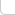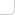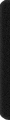# 某岛

… : "…アッカリ～ン . .. . " .. .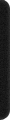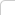June 21, 2012

## 主席树学习笔记 Lv. 1

。。总之由于原名字太长了，而且 “主席” 两个字念起来冷艳高贵，以后全部称之为主席树好了。。。

• 线段树的每个结点，保存的是这个区间含有的数字的个数。
• 主席树的每个结点，也就是每颗线段树的大小和形态也是一样的，也就是主席树之间可以相互进行加减运算。。

• 主席树是一种离线结构。。（必须预先知道所有数字的范围。。这在一些应用中会成为障碍。。。
• 存在 MLE 问题。。（如果按照定义里面的方法去写，对每个结点都需要开一整可线段树，至少都是 O(n2) 级别的空间。。

——————————————————

const int N = 50009, M = 10009, NN = 2500009;

int l[NN], r[NN], c[NN], total;

PII A[N+M]; int B[N+M], Q[M];
int S[N], C[N], Null;
int n, m, An, Tn;
.. .


l[], r[], c[], total 分别是左孩子下标，右孩子下标，该区间的数字个数和当前已分配的结点数。
（线段树的区间可以在递归的时候实时计算，可以不予保存。。。

PII A[]; int B[]; A、B 用于离散化。。。 B 记录相应数字的 Rank 值。
int Q[]; 记录询问。。。

int S[], C[], Null; 是主席树下标。。

An 是 A 数组的长度，由初始 n 个数字和修改后的数字决定，
Tn 是所有出现的不同数字的个数，用于建立线段树，由对 A 数组去重后得到。

#define lx l[x]
#define rx r[x]
#define ly l[y]
#define ry r[y]
#define cx c[x]
#define cy c[y]

#define mid ((ll+rr)>>1)
#define lc lx, ll, mid
#define rc rx, mid+1, rr

void Build(int &x, int ll, int rr){
x = ++total; if (ll < rr) Build(lc), Build(rc);
}

int Insert(int y, int p, int d){

int x = ++total, root = x;

c[x] = c[y] + d; int ll = 0, rr = Tn;

while (ll < rr){
if (p <= mid){
lx = ++total, rx = ry;
x = lx, y = ly, rr = mid;
}
else {
lx = ly, rx = ++total;
x = rx, y = ry, ll = mid + 1;
}
c[x] = c[y] + d;
}

return root;
}


。。。没有修改的链仍然指向 y 的相对应部分。复杂度为树高。。。。

。。。下面是 int main(); 函数代码了。。。完整程序移步这里。。。。

int main(){

#ifndef ONLINE_JUDGE
freopen("in.txt", "r", stdin);
//freopen("out.txt", "w", stdout);
#endif

#define key first
#define id second

RD(n, m); REP(i, n) A[i] = MP(RD(), i);

An = n; char cmd; REP(i, m){
RC(cmd); if(cmd == 'Q') RD(Q[i], Q[i], Q[i]);
else RD(Q[i]), Q[i] = 0, A[An++] = MP(RD(), An);
}

sort(A, A + An), B[A.id] = Tn = 0;
FOR(i, 1, An){
if(A[i].key != A[i-1].key) A[++Tn].key = A[i].key;
B[A[i].id] = Tn;
}

Build(Null, 0, Tn); REP_1(i, n) C[i] = Null;

S = Null; REP(i, n){
S[i+1] = Insert(S[i], B[i], 1);
}

An = n;

REP(i, m) if (Q[i]){
OT(A[Query(Q[i], Q[i], Q[i])].key);
}else{
Modify(Q[i], B[Q[i]-1], -1);
Modify(Q[i], B[Q[i]-1] = B[An++], 1);
}
}


1. 由于每棵线段树的大小形态都是一样的，而且初始值全都是 0，那每个线段树都初始化不是太浪费了？所以一开始只要建一棵空树即可。

事实上由于当一个结点的 c[] 结果为 0 时。。无论它向左还是向右递归下去结果都会是0 。。。
所以初始时甚至不是建立空树。。而是只需要一个空结点即可。。。（如何实现这层。。。

2. 仍然没有将 Lazy Evalutaion 的理念做到完美。。。这题中的 Lazy Evaluation 体现在两个方面。。
主席树的单链修改和主席树与主席树之间的运算。。

主席树与主席树之间的运算如果全部做的话一次是 O(n)。。。但是因为询问时只需要计算一条到叶子的路径。。。
。。。上面的代码中，这种运算是通过 Struct Pack; 结构体现的。。。一种多颗主席树结点的打包。。
。。全部在同一层的同一个位置。。向同一个方向递归下降。。一个 Pack; 的值为该结构内所有结点值的和。。

。。现在实现的不足之处：

• 无法很好的处理减运算。。因此这里才还要保存了 S[] 前缀和，让 C[] 逐个逐个修改。。。
。。。然后每次回答询问的时候还要一并带上 S[] 。。Orz。（虽然说有助于预处理。。
• 对计算过后结果无法有效利用。。（换句话说没有体现出主席式 Memorization 的一面。。。
• 对单个链的插入操作也不是要即时处理的。。（。。如何用数据结构把所有操作先 Hold 下来 。。?。。这样的好处是有一些操作对结果没有影响是可以忽略。。另外把一堆操作放在一起当做一个操作处理的话有时也能得到 Bonus 。。。
3. Fotile 大神说如果加上垃圾回收的话，可以去掉一个 log…… ym

（另外正一个误。。。加上回收并不能去掉一个 log 。。。。